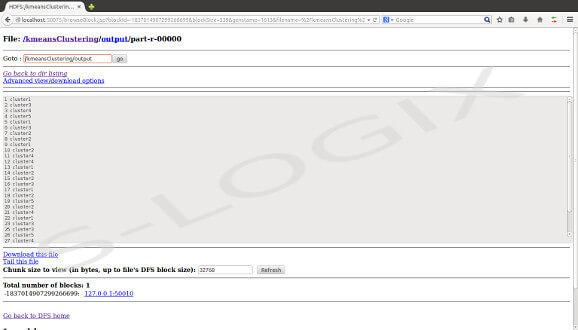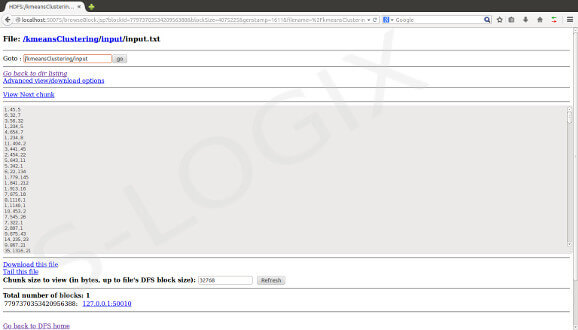• #5, First Floor, 4th Street Dr. Subbarayan Nagar Kodambakkam, Chennai-600 024 Landmark : Samiyar Madam
• pro@slogix.in
• +91- 81240 01111

#### Description

The K-means clustering algorithm groups similar objects into number of clusters. It refines the cluster center point iteratively until the maximum intra cluster deviation is reached. Map Reduce Framework is implemented to cluster large data points.. The below source code process the input file that consists of data points and initial center points. In mapper class the Map function reads this file to get the centers from centers.txt file. Then it reads the input rows (the data) in input.txt file and calculates the distance to each center. By processing each row, it produces an output pair with key as cluster id and value as the coordinates of row. After the completion of shuffle and sort phase, the output is transformed into reducer. In Reducer class, the reduce function calculates the mean value of coordinates belongs to each cluster and the mean values are updated as new centers in centers.txt file.

###### Sample Code

Mapper Function

//Map function find nearest centers to the coordinates

Map Phase input:<k1, v1>

k1- line number

v1- point(coordinates)

//Find minimum center from point

For each center

Find minimum distance to point

End for

k2-nearest center

V2-point

Output: <k2,v2>

Reducer Function

//Compute new cluster centers

Reduce Phase input:<k2, List<v2>>

Calculate mean value for v2 points

new center point-mean value

k3-new center point

v3-points

Output: <k3,v3>

Continue the process till the clusters are converged.

###### Screenshots

input

Output

First column – Document id

Second column – cluster id